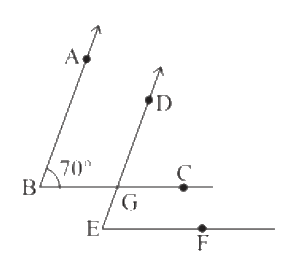# Ex.5.2 Q5 Lines and Angles - NCERT Maths Class 7

Go back to  'Ex.5.2'

## Question

In the given figure, the arms of two angles are parallel. If $$\angle ABC = 70^\circ,$$ then find:

(i) $$\angle DGC$$

(ii) $$\angle DEF$$Video Solution
Lines & Angles
Ex 5.2 | Question 5

## Text Solution

Reasoning:

Let’s visually model this problem. There is one operation that can be done. According to this model, the resultant value of corresponding angle will be equal to $$\angle DGC.$$

Now, it’s a matter of finding measure of $$\angle DGC.$$

Steps:

(i) Solve for $$\angle DGC$$

Given $$AB || DG$$ and $$BC$$ is transversal

Also,

$$\angle ABC = 70^\circ\text{ (Given)}$$

Since,

$$\angle ABC = \angle DGC\text{(Corresponding angles)}$$

Therefore$$, \angle DGC = 70^\circ→(1)$$

Reasoning:

Let’s visually model this problem . There is one operation that can be done.According to this model, the resultant value of corresponding angle will be equal to $$\angle DGC.$$ Now, it’s a matter of finding measure of $$\angle DEF.$$

Steps:

(ii) Solve for $$\angle DEF$$

Given $$BC || EF$$ and $$DE$$ is transversal

Also, $$\angle DGC = 70^\circ \text{(from 1)}$$

Since,

$$\angle DGC = \angle DEF \text{(Corresponding angles)}$$

Therefore$$, \angle DEF = 70^\circ$$

Learn from the best math teachers and top your exams

• Live one on one classroom and doubt clearing
• Practice worksheets in and after class for conceptual clarity
• Personalized curriculum to keep up with school